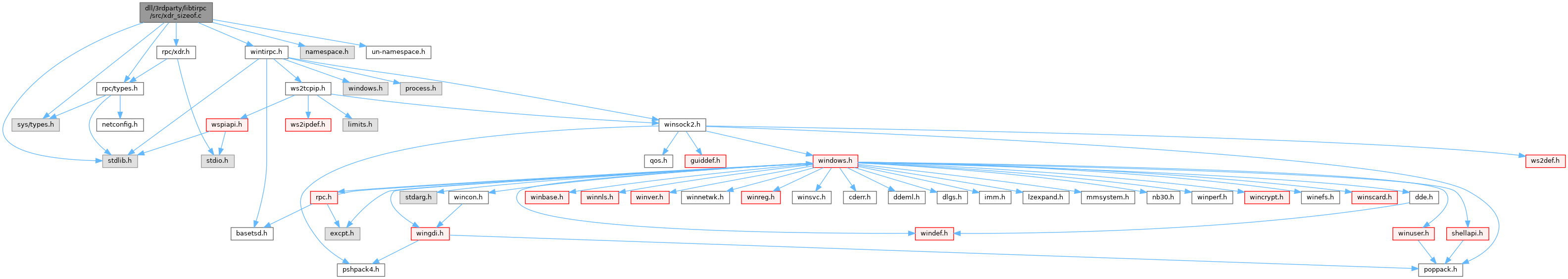ReactOS 0.4.15-dev-6657-ged9973f
xdr_sizeof.c File Reference
`#include <wintirpc.h>`
`#include "namespace.h"`
`#include <rpc/types.h>`
`#include <rpc/xdr.h>`
`#include <sys/types.h>`
`#include <stdlib.h>`
`#include "un-namespace.h"`
Include dependency graph for xdr_sizeof.c:Go to the source code of this file.

## Functions

static bool_t x_putlong (XDR *xdrs, const long *longp)

static bool_t x_putbytes (XDR *xdrs, const char *bp, u_int len)

static u_int x_getpostn (XDR *xdrs)

static bool_t x_setpostn (XDR *xdrs, u_int pos)

static int32_tx_inline (XDR *xdrs, u_int len)

static int harmless ()

static void x_destroy (XDR *xdrs)

unsigned long xdr_sizeof (xdrproc_t func, void *data)

## ◆ harmless()

 static int harmless ( )
static

Definition at line 106 of file xdr_sizeof.c.

107{
108 /* Always return FALSE/NULL, as the case may be */
109 return (0);
110}

Referenced by xdr_sizeof().

## ◆ x_destroy()

 static void x_destroy ( XDR * xdrs )
static

Definition at line 113 of file xdr_sizeof.c.

114{
115 xdrs->x_handy = 0;
116 xdrs->x_base = 0;
117 if (xdrs->x_private) {
118 free(xdrs->x_private);
119 xdrs->x_private = NULL;
120 }
121 return;
122}
#define free
Definition: debug_ros.c:5
#define NULL
Definition: types.h:112
char * x_base
Definition: xdr.h:126
void * x_private
Definition: xdr.h:125
u_int x_handy
Definition: xdr.h:127

Referenced by xdr_sizeof().

## ◆ x_getpostn()

 static u_int x_getpostn ( XDR * xdrs )
static

Definition at line 64 of file xdr_sizeof.c.

65{
66 return (xdrs->x_handy);
67}

Referenced by xdr_sizeof().

## ◆ x_inline()

 static int32_t * x_inline ( XDR * xdrs, u_int len )
static

Definition at line 78 of file xdr_sizeof.c.

79{
80 if (len == 0) {
81 return (NULL);
82 }
83 if (xdrs->x_op != XDR_ENCODE) {
84 return (NULL);
85 }
86 if (len < PtrToUlong(xdrs->x_base)) {
87 /* x_private was already allocated */
88 xdrs->x_handy += len;
89 return ((int32_t *) xdrs->x_private);
90 } else {
91 /* Free the earlier space and allocate new area */
92 if (xdrs->x_private)
93 free(xdrs->x_private);
94 if ((xdrs->x_private = (caddr_t) malloc(len)) == NULL) {
95 xdrs->x_base = 0;
96 return (NULL);
97 }
98 xdrs->x_base = UIntToPtr(len);
99 //xdrs->x_base = len; // XXX Is this right???
100 xdrs->x_handy += len;
101 return ((int32_t *) xdrs->x_private);
102 }
103}
#define UIntToPtr(ui)
Definition: basetsd.h:90
#define malloc
Definition: debug_ros.c:4
INT32 int32_t
Definition: types.h:71
#define PtrToUlong(u)
Definition: config.h:107
GLenum GLsizei len
Definition: glext.h:6722
Definition: rosdhcp.h:36
enum xdr_op x_op
Definition: xdr.h:104
@ XDR_ENCODE
Definition: xdr.h:85

Referenced by xdr_sizeof().

## ◆ x_putbytes()

 static bool_t x_putbytes ( XDR * xdrs, const char * bp, u_int len )
static

Definition at line 57 of file xdr_sizeof.c.

58{
59 xdrs->x_handy += len;
60 return (TRUE);
61}
#define TRUE
Definition: types.h:120

Referenced by xdr_sizeof().

## ◆ x_putlong()

 static bool_t x_putlong ( XDR * xdrs, const long * longp )
static

Definition at line 49 of file xdr_sizeof.c.

50{
52 return (TRUE);
53}
#define BYTES_PER_XDR_UNIT
Definition: xdr.h:93

Referenced by xdr_sizeof().

## ◆ x_setpostn()

 static bool_t x_setpostn ( XDR * xdrs, u_int pos )
static

Definition at line 71 of file xdr_sizeof.c.

72{
73 /* This is not allowed */
74 return (FALSE);
75}
#define FALSE
Definition: types.h:117

Referenced by xdr_sizeof().

## ◆ xdr_sizeof()

 unsigned long xdr_sizeof ( xdrproc_t func, void * data )

Definition at line 125 of file xdr_sizeof.c.

128{
129 XDR x;
130 struct xdr_ops ops;
131 bool_t stat;
132 /* to stop ANSI-C compiler from complaining */
133 typedef bool_t (* dummyfunc1)(XDR *, long *);
134 typedef bool_t (* dummyfunc2)(XDR *, caddr_t, u_int);
135
136 ops.x_putlong = x_putlong;
137 ops.x_putbytes = x_putbytes;
138 ops.x_inline = x_inline;
139 ops.x_getpostn = x_getpostn;
140 ops.x_setpostn = x_setpostn;
141 ops.x_destroy = x_destroy;
142
143 /* the other harmless ones */
144 ops.x_getlong = (dummyfunc1) harmless;
145 ops.x_getbytes = (dummyfunc2) harmless;
146
147 x.x_op = XDR_ENCODE;
148 x.x_ops = &ops;
149 x.x_handy = 0;
152
153 stat = func(&x, data);
154 if (x.x_private)
155 free(x.x_private);
156 return (stat == TRUE ? (unsigned) x.x_handy: 0);
157}
#define stat
Definition: acwin.h:99
Definition: ftp.c:24
UINT32 u_int
Definition: types.h:82
int32_t bool_t
Definition: types.h:101
GLint GLint GLint GLint GLint x
Definition: gl.h:1548
GLint GLenum GLsizei GLsizei GLsizei GLint GLsizei const GLvoid * data
Definition: gl.h:1950
GLenum func
Definition: glext.h:6028
Definition: xdr.h:103
Definition: stat.h:55
static bool_t x_putlong(XDR *xdrs, const long *longp)
Definition: xdr_sizeof.c:49
static bool_t x_setpostn(XDR *xdrs, u_int pos)
Definition: xdr_sizeof.c:71
static bool_t x_putbytes(XDR *xdrs, const char *bp, u_int len)
Definition: xdr_sizeof.c:57
static int32_t * x_inline(XDR *xdrs, u_int len)
Definition: xdr_sizeof.c:78
static int harmless()
Definition: xdr_sizeof.c:106
static void x_destroy(XDR *xdrs)
Definition: xdr_sizeof.c:113
static u_int x_getpostn(XDR *xdrs)
Definition: xdr_sizeof.c:64